### Learning Objectives

• Find the derivative of a complicated function by using implicit differentiation.
• Use implicit differentiation to determine the equation of a tangent line.

We have already studied how to find equations of tangent lines to functions and the rate of change of a function at a specific point. In all these cases we had the explicit equation for the function and differentiated these functions explicitly. Suppose instead that we want to determine the equation of a tangent line to an arbitrary curve or the rate of change of an arbitrary curve at a point. In this section, we solve these problems by finding the derivatives of functions that define $y$ implicitly in terms of $x$.

# Implicit Differentiation

In most discussions of math, if the dependent variable $y$ is a function of the independent variable $x$, we express $y$ in terms of $x$. If this is the case, we say that $y$ is an explicit function of $x$. For example, when we write the equation $y=x^2+1$, we are defining $y$ explicitly in terms of $x$. On the other hand, if the relationship between the function $y$ and the variable $x$ is expressed by an equation where $y$ is not expressed entirely in terms of $x$, we say that the equation defines $y$ implicitly in terms of $x$. For example, the equation $y-x^2=1$ defines the function $y=x^2+1$ implicitly.

Implicit differentiation allows us to find slopes of tangents to curves that are clearly not functions (they fail the vertical line test). We are using the idea that portions of $y$ are functions that satisfy the given equation, but that $y$ is not actually a function of $x$.

In general, an equation defines a function implicitly if the function satisfies that equation. An equation may define many different functions implicitly. For example, the functions

$y=\sqrt{25-x^2}$, $y = -\sqrt{25-x^2}$, and $y=\begin{cases} \sqrt{25-x^2} & \text{if} \, -5 \le x < 0 \\ -\sqrt{25-x^2} & \text{if} \, 0 \le x \le 5 \end{cases}$, which are illustrated in (Figure) , are just three of the many functions defined implicitly by the equation $x^2+y^2=25$.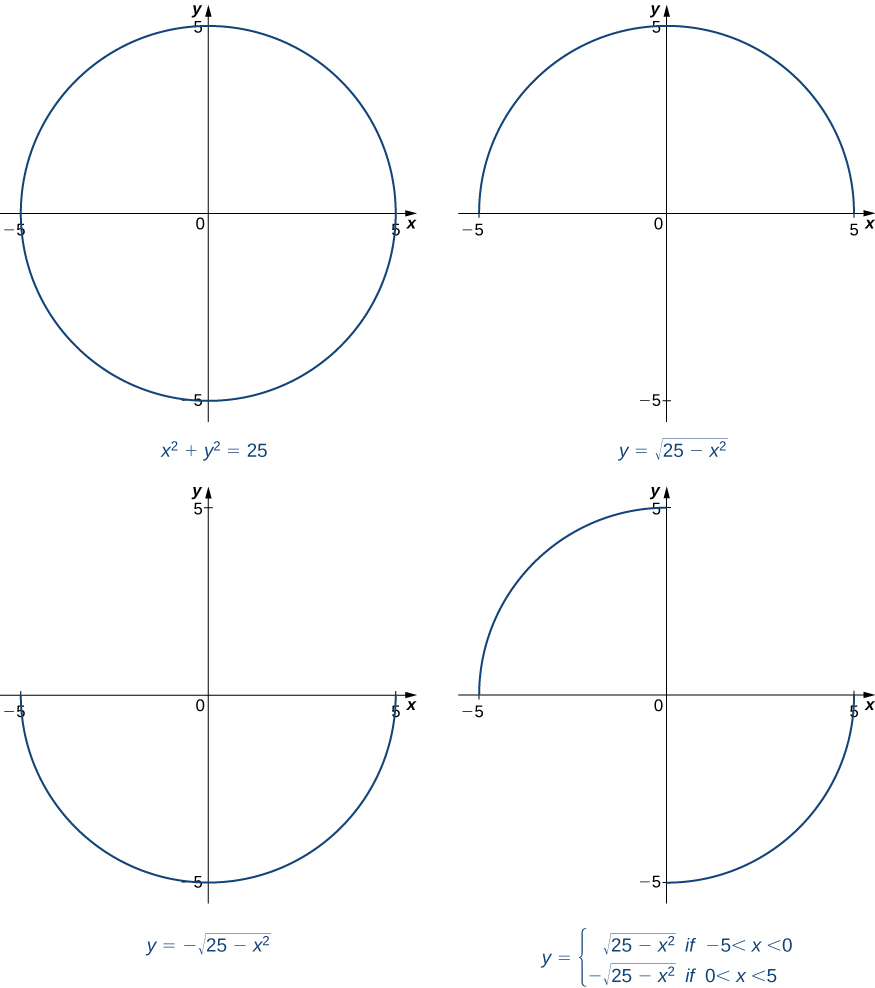Figure 1. The equation ${x}^{2}+{y}^{2}=25$ defines many functions implicitly.

If we want to find the slope of the line tangent to the graph of $x^2+y^2=25$ at the point $(3,4)$, we could evaluate the derivative of the function $y=\sqrt{25-x^2}$ at $x=3$. On the other hand, if we want the slope of the tangent line at the point $(3,-4)$, we could use the derivative of $y=-\sqrt{25-x^2}$. However, it is not always easy to solve for a function defined implicitly by an equation. Fortunately, the technique of implicit differentiation allows us to find the derivative of an implicitly defined function without ever solving for the function explicitly. The process of finding $\frac{dy}{dx}$ using implicit differentiation is described in the following problem-solving strategy.

### Problem-Solving Strategy: Implicit Differentiation

To perform implicit differentiation on an equation that defines a function $y$ implicitly in terms of a variable $x$, use the following steps:

1. Take the derivative of both sides of the equation. Keep in mind that $y$ is a function of $x$. Consequently, whereas $\frac{d}{dx}(\sin x)= \cos x, \, \frac{d}{dx}(\sin y)= \cos y\frac{dy}{dx}$ because we must use the Chain Rule to differentiate $\sin y$ with respect to $x$.
2. Rewrite the equation so that all terms containing $\frac{dy}{dx}$ are on the left and all terms that do not contain $\frac{dy}{dx}$ are on the right.
3. Factor out $\frac{dy}{dx}$ on the left.
4. Solve for $\frac{dy}{dx}$ by dividing both sides of the equation by an appropriate algebraic expression.

### Using Implicit Differentiation

Assuming that $y$ is defined implicitly by the equation $x^2+y^2=25$, find $\frac{dy}{dx}$.

#### Solution

Follow the steps in the problem-solving strategy.

$\begin{array}{llll} \frac{d}{dx}(x^2+y^2) = \frac{d}{dx}(25) & & & \text{Step 1. Differentiate both sides of the equation.} \\ \frac{d}{dx}(x^2)+\frac{d}{dx}(y^2) = 0 & & & \begin{array}{l}\text{Step 1.1. Use the sum rule on the left.} \\ \text{On the right,} \, \frac{d}{dx}(25)=0. \end{array} \\ 2x+2y\frac{dy}{dx} = 0 & & & \begin{array}{l}\text{Step 1.2. Take the derivatives, so} \, \frac{d}{dx}(x^2)=2x \\ \text{and} \, \frac{d}{dx}(y^2)=2y\frac{dy}{dx}. \end{array} \\ 2y\frac{dy}{dx} = -2x & & & \begin{array}{l}\text{Step 2. Keep the terms with} \, \frac{dy}{dx} \, \text{on the left.} \\ \text{Move the remaining terms to the right.} \end{array} \\ \frac{dy}{dx} = -\frac{x}{y} & & & \begin{array}{l}\text{Step 4. Divide both sides of the equation by} \\ 2y. \, \text{(Step 3 does not apply in this case.)} \end{array} \end{array}$

#### Analysis

Note that the resulting expression for $\frac{dy}{dx}$ is in terms of both the independent variable $x$ and the dependent variable $y$. Although in some cases it may be possible to express $\frac{dy}{dx}$ in terms of $x$ only, it is generally not possible to do so.

### Using Implicit Differentiation and the Product Rule

Assuming that $y$ is defined implicitly by the equation $x^3 \sin y+y=4x+3$, find $\frac{dy}{dx}$.

#### Solution

$\begin{array}{llll}\frac{d}{dx}(x^3 \sin y+y) = \frac{d}{dx}(4x+3) & & & \text{Step 1: Differentiate both sides of the equation.} \\ \frac{d}{dx}(x^3 \sin y)+\frac{d}{dx}(y) = 4 & & & \begin{array}{l}\text{Step 1.1: Apply the sum rule on the left.} \\ \text{On the right,} \, \frac{d}{dx}(4x+3)=4. \end{array} \\ (\frac{d}{dx}(x^3) \cdot \sin y+\frac{d}{dx}(\sin y) \cdot x^3) + \frac{dy}{dx} = 4 & & & \begin{array}{l}\text{Step 1.2: Use the product rule to find} \\ \frac{d}{dx}(x^3 \sin y). \, \text{Observe that} \, \frac{d}{dx}(y)=\frac{dy}{dx}. \end{array} \\ 3x^2 \sin y+(\cos y\frac{dy}{dx}) \cdot x^3 + \frac{dy}{dx} = 4 & & & \begin{array}{l}\text{Step 1.3: We know} \, \frac{d}{dx}(x^3)=3x^2. \, \text{Use the} \\ \text{chain rule to obtain} \, \frac{d}{dx}(\sin y)= \cos y\frac{dy}{dx}. \end{array} \\ x^3 \cos y\frac{dy}{dx}+\frac{dy}{dx} = 4-3x^2 \sin y & & & \begin{array}{l}\text{Step 2: Keep all terms containing} \, \frac{dy}{dx} \, \text{on the} \\ \text{left. Move all other terms to the right.} \end{array} \\ \frac{dy}{dx}(x^3 \cos y+1) = 4-3x^2 \sin y & & & \text{Step 3: Factor out} \, \frac{dy}{dx} \, \text{on the left.} \\ \frac{dy}{dx} = \large \frac{4-3x^2 \sin y}{x^3 \cos y+1} & & & \begin{array}{l}\text{Step 4: Solve for} \, \frac{dy}{dx} \, \text{by dividing both sides of} \\ \text{the equation by} \, x^3 \cos y+1. \end{array} \end{array}$

### Using Implicit Differentiation to Find a Second Derivative

Find $\frac{d^2 y}{dx^2}$ if $x^2+y^2=25$.

#### Solution

In (Figure) , we showed that $\frac{dy}{dx}=-\frac{x}{y}$. We can take the derivative of both sides of this equation to find $\frac{d^2 y}{dx^2}$.

$\begin{array}{lllll} \frac{d^2 y}{dx^2} & =\large \frac{d}{dx}(-\frac{x}{y}) & & & \text{Differentiate both sides of} \, \frac{dy}{dx}=-\frac{x}{y}. \\ & = \large -\frac{(1 \cdot y-x\frac{dy}{dx})}{y^2} & & & \text{Use the quotient rule to find} \, \frac{d}{dx}(-\frac{x}{y}). \\ & = \large \frac{-y+x\frac{dy}{dx}}{y^2} & & & \text{Simplify.} \\ & = \large \frac{-y+x(-\frac{x}{y})}{y^2} & & & \text{Substitute} \, \frac{dy}{dx}=-\frac{x}{y}. \\ & = \large \frac{-y^2-x^2}{y^3} & & & \text{Simplify.} \end{array}$

At this point we have found an expression for $\frac{d^2 y}{dx^2}$. If we choose, we can simplify the expression further by recalling that $x^2+y^2=25$ and making this substitution in the numerator to obtain $\frac{d^2 y}{dx^2}=-\frac{25}{y^3}$.

Find $\frac{dy}{dx}$ for $y$ defined implicitly by the equation $4x^5+ \tan y=y^2+5x$.

#### Hint

Follow the problem solving strategy, remembering to apply the chain rule to differentiate $\tan y$ and $y^2$.

#### Solution

$\frac{dy}{dx}=\large \frac{5-20x^4}{\sec^2 y-2y}$

# Finding Tangent Lines Implicitly

Now that we have seen the technique of implicit differentiation, we can apply it to the problem of finding equations of tangent lines to curves described by equations.

### Finding a Tangent Line to a Circle

Find the equation of the line tangent to the curve $x^2+y^2=25$ at the point $(3,-4)$.

#### Solution

Although we could find this equation without using implicit differentiation, using that method makes it much easier. In (Figure) , we found $\frac{dy}{dx}=-\frac{x}{y}$.

The slope of the tangent line is found by substituting $(3,-4)$ into this expression. Consequently, the slope of the tangent line is $\frac{dy}{dx}|_{(3,-4)} =-\frac{3}{-4}=\frac{3}{4}$.

Using the point $(3,-4)$ and the slope $\frac{3}{4}$ in the point-slope equation of the line, we then solve for $y$ to obtain the equation $y=\frac{3}{4}x-\frac{25}{4}$ ( (Figure) ).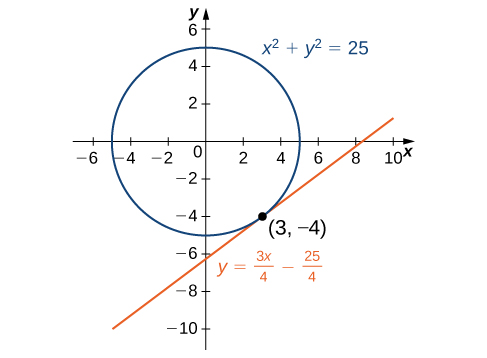Figure 2. The line $y=\frac{3}{4}x-\frac{25}{4}$ is tangent to $x^2+y^2=25$ at the point $(3,-4)$.

### Finding the Equation of the Tangent Line to a Curve

Find the equation of the line tangent to the graph of $y^3+x^3-3xy=0$ at the point $(\frac{3}{2},\frac{3}{2})$ ( (Figure) ). This curve is known as the folium (or leaf) of Descartes.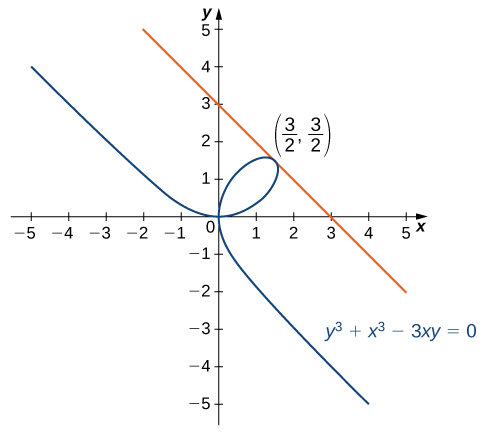Figure 3. Finding the tangent line to the folium of Descartes at $(\frac{3}{2},\frac{3}{2})$.

#### Solution

Begin by finding $\frac{dy}{dx}.$

$\begin{array}{l} \frac{d}{dx}(y^3+x^3-3xy) = \frac{d}{dx}(0) \\ \\ 3y^2\frac{dy}{dx}+3x^2-(3y+3x\frac{dy}{dx}) = 0 \\ \\ \frac{dy}{dx} = \frac{3y-3x^2}{3y^2-3x}. \\ \\ \end{array}$

Next, substitute $(\frac{3}{2},\frac{3}{2})$ into $\frac{dy}{dx}=\frac{3y-3x^2}{3y^2-3x}$ to find the slope of the tangent line:

$\frac{dy}{dx}|_{(\frac{3}{2},\frac{3}{2})}=-1$.

Finally, substitute into the point-slope equation of the line and solve for $y$ to obtain

$y=-x+3$.

### Applying Implicit Differentiation

In a simple video game, a rocket travels in an elliptical orbit whose path is described by the equation $4x^2+25y^2=100$. The rocket can fire missiles along lines tangent to its path. The object of the game is to destroy an incoming asteroid traveling along the positive $x$-axis toward $(0,0)$. If the rocket fires a missile when it is located at $(3,\frac{8}{3})$, where will it intersect the $x$-axis?

#### Solution

To solve this problem, we must determine where the line tangent to the graph of

$4x^2+25y^2=100$ at $(3,\frac{8}{3})$ intersects the $x$-axis. Begin by finding $\frac{dy}{dx}$ implicitly.

Differentiating, we have

$8x+50y\frac{dy}{dx}=0$.

Solving for $\frac{dy}{dx}$, we have

$\frac{dy}{dx}=-\frac{4x}{25y}$.

The slope of the tangent line is $\frac{dy}{dx}|_{(3,\frac{8}{3})}=-\frac{9}{50}$. The equation of the tangent line is $y=-\frac{9}{50}x+\frac{481}{150}$. To determine where the line intersects the $x$-axis, solve $0=-\frac{9}{50}x+\frac{481}{150}$. The solution is $x=\frac{481}{27}$. The missile intersects the $x$-axis at the point $(\frac{481}{27},0)$.

Find the equation of the line tangent to the hyperbola $x^2-y^2=16$ at the point $(5,3)$.

#### Hint

Using implicit differentiation, you should find that $\frac{dy}{dx}=\frac{x}{y}$.

#### Solution

$y=\frac{5}{3}x-\frac{16}{3}$

### Key Concepts

• We use implicit differentiation to find derivatives of implicitly defined functions (functions defined by equations).
• By using implicit differentiation, we can find the equation of a tangent line to the graph of a curve.

For the following exercises, use implicit differentiation to find $\frac{dy}{dx}$.

1.  $x^2-y^2=4$

2.  $6x^2+3y^2=12$

#### Solution

$\frac{dy}{dx}=\frac{-2x}{y}$

3.  $x^2 y=y-7$

4.  $3x^3+9xy^2=5x^3$

#### Solution

$\frac{dy}{dx}=\frac{x}{3y}-\frac{y}{2x}$

5.  $xy- \cos (xy)=1$

6.  $y\sqrt{x+4}=xy+8$

#### Solution

$\frac{dy}{dx}=\large \frac{y-\frac{y}{2\sqrt{x+4}}}{\sqrt{x+4}-x}$

7.  $-xy-2=\frac{x}{7}$

8.  $y \sin(xy)=y^2+2$

#### Solution

$\frac{dy}{dx}=\large \frac{y^2 \cos(xy)}{2y- \sin(xy)-xy \cos xy}$

9.  $(xy)^2+3x=y^2$

10.  $x^3 y+xy^3=-8$

$\frac{dy}{dx}=\large \frac{-3x^2 y-y^3}{x^3+3xy^2}$

11. $x^2y+xy^2 = 3x$

12. $e^{x^2y} = x+ y$

$\frac{dy}{dx}=\large \frac{1-2xye^{x^2y}}{x^2e^{x^2y}-1}$

For the following exercises, find $y''(x)$ using implicit differentiation. Leave your final answer in terms of $x$ and $y$ only.

13. $x^3 + y ^3 = 1$.

14. $x^2 +y^2 = 2$

$y''(x)=\large \frac{-y - \frac{x^2}{y}}{y^2} = \frac{-(y^2 + x^2)}{y^3}$

15. $-xy^2+3y = 5$

16. $4x = xy + 2y$

$y''(x)=\large \frac{(4-\frac{4-y}{x+2}) (x+2) - (4-y)}{(x+2)^2}$

For the following exercises, find the equation of the tangent line to the graph of the given equation at the indicated point. Use a calculator or computer software to graph the function and the tangent line.

17. [T]  $x^4 y-xy^3=-2, \, (-1,-1)$

18. [T]  $x^2 y^2+5xy=14, \, (2,1)$

#### Solution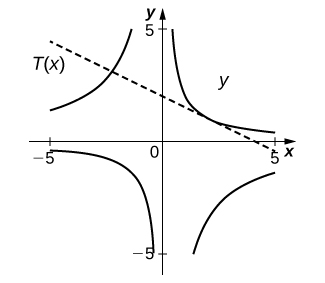$y=-\frac{1}{2}x+2$

19. [T]  $\tan (xy)=y, \, (\frac{\pi}{4},1)$

20. [T]  $xy^2 + \sin(\pi y)-2x^2=10, \, (2,-3)$

#### Solution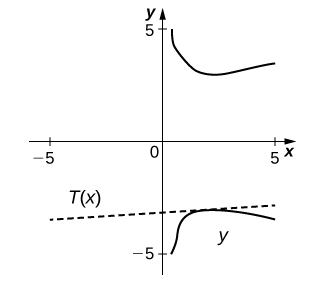$y=\large \frac{1}{\pi +12}x-\frac{3\pi +38}{\pi +12}$

21. [T]  $\frac{x}{y}+5x-7=-\frac{3}{4}y, \, (1,2)$

22. [T]  $xy+ \sin (x)=1, \, (\frac{\pi}{2},0)$

#### Solution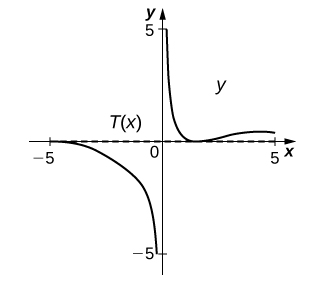$y=0$

23. [T] The graph of a folium of Descartes with equation $2x^3+2y^3-9xy=0$ is given in the following graph.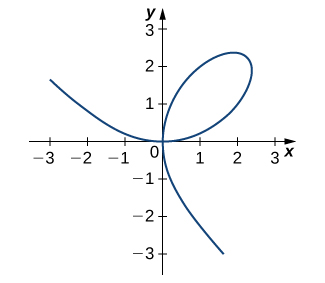1. Find the equation of the tangent line at the point $(2,1)$. Graph the tangent line along with the folium.
2. Find the equation of the normal line to the tangent line in a. at the point $(2,1)$.

24.  For the equation $x^2+2xy-3y^2=0$,

1. Find the equation of the normal to the tangent line at the point $(1,1)$.
2. At what other point does the normal line in a. intersect the graph of the equation?

#### Solution

a. $y=-x+2$
b. $(3,-1)$

25.  Find all points on the graph of $y^3-27y=x^2-90$ at which the tangent line is vertical.

26.  For the equation $x^2+xy+y^2=7$,

1. Find the $x$-intercept(s).
2. Find the slope of the tangent line(s) at the $x$-intercept(s).
3. What does the value(s) in b. indicate about the tangent line(s)?

#### Solution

a. $(\pm \sqrt{7},0)$
b. Slope is -2 at both intercepts
c. They are parallel since the slope is the same at both intercepts.

27.  Find the equation of the tangent line to the graph of the equation $\sin^{-1} x+\sin^{-1} y=\frac{\pi}{6}$ at the point $(0,\frac{1}{2})$.

28.  Find the equation of the tangent line to the graph of the equation $\tan^{-1}(x+y)=x^2+\frac{\pi}{4}$ at the point $(0,1)$.

#### Solution

$y=-x+1$

29.  Find $y^{\prime}$ and $y''$ for $x^2+6xy-2y^2=3$.

30. [T] The number of cell phones produced when $x$ dollars is spent on labor and $y$ dollars is spent on capital invested by a manufacturer can be modeled by the equation $60x^{3/4}y^{1/4}=3240$.

1. Find $\frac{dy}{dx}$ and evaluate at the point $(81,16)$.
2. Interpret the result of a.

#### Solution

a. -0.5926
b. When $81 is spent on labor and$16 is spent on capital, the amount spent on capital is decreasing by $0.5926 per$1 spent on labor.

31. [T] The number of cars produced when $x$ dollars is spent on labor and $y$ dollars is spent on capital invested by a manufacturer can be modeled by the equation $30x^{1/3}y^{2/3}=360$.

(Both $x$ and $y$ are measured in thousands of dollars.)

1. Find $\frac{dy}{dx}$ and evaluate at the point $(27,8)$.
2. Interpret the result of a.

32.  The volume of a right circular cone of radius $x$ and height $y$ is given by $V=\frac{1}{3}\pi x^2 y$. Suppose that the volume of the cone is $85\pi \, \text{cm}^3$. Find $\frac{dy}{dx}$ when $x=4$ and $y=16$.

#### Solution

$\frac{dy}{dx}=-8$

For the following exercises, consider a closed rectangular box with a square base with side $x$ and height $y$.

33.  Find an equation for the surface area of the rectangular box, $S(x,y)$.

34.  If the surface area of the rectangular box is 78 square feet, find $\frac{dy}{dx}$ when $x=3$ feet and $y=5$ feet.

#### Solution

$\frac{dy}{dx}=-2.67$

For the following exercises, use implicit differentiation to determine $y^{\prime}$. Does the answer agree with the formulas we have previously determined?

35.  $x= \sin y$

36.  $x= \cos y$

#### Solution

$y^{\prime}=-\frac{1}{\sqrt{1-x^2}}$

37.  $x= \tan y$

## Glossary

implicit differentiation
is a technique for computing $\frac{dy}{dx}$ for a function defined by an equation, accomplished by differentiating both sides of the equation (remembering to treat the variable $y$ as a function) and solving for $\frac{dy}{dx}$